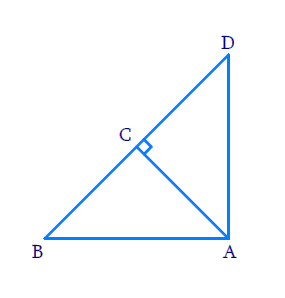# Ex.6.5 Q3 Triangles Solution - NCERT Maths Class 10

Go back to  'Ex.6.5'

## Question

In Figure, $${ABD}$$ is a triangle right angled at $$A$$ and $${AC⊥BD}$$. Show that

(i) $$A{{B}^{2}}=\text{ }BC.\text{ }BD$$

(ii) $$A{{C}^{2}}=\text{ }BC.\text{ }DC$$

(iii) $$A{{D}^{2}}=\text{ }BD.\text{ }CD$$

Diagram## Text Solution

Reasoning:

As we know if a perpendicular is drawn from the vertex of the right angle of a right triangle to the hypotenuse then triangles on both sides of the perpendicular are similar to the whole triangle and to each other.

Steps:

i). In $$\Delta BAD\;\text{and}\;\Delta BCA$$

$\begin{array}{l} \Rightarrow \,\,\Delta BAD \sim \Delta BCA\\ \Rightarrow \frac{{AB}}{{BC}} = \frac{{BD}}{{AB}}{\rm{ }}\qquad\left( {{\text{Corresponding sides of similar triangle}}} \right)\\ \Rightarrow A{B^2} = BC \cdot BD \end{array}$

ii). In $$\Delta BCA\,\,\text{and}\,\Delta ACD$$

$\begin{array}{l} \Rightarrow \,\Delta BCA \sim \Delta ACD\\ \, \Rightarrow \,\frac{{AC}}{{CD}} = \frac{{BC}}{{AC}}{\rm{ }}\qquad\;\left( {{\text{Corresponding sides of similar triangle}}} \right)\\ \Rightarrow \,A{C^2} = BC \cdot DC \end{array}$

iii). In $$\Delta BAD$$ and $$\Delta ACD$$

$\begin{array}{l} \Rightarrow \Delta BAD \sim \Delta ACD\\ \Rightarrow \frac{{AD}}{{CD}} = \frac{{BD}}{{AD}}{\rm{ }}\qquad\;\left( {{\text{Corresponding sides of similar triangle}}} \right)\\ \,\,\,\,\,A{D^2} = BD \cdot CD \end{array}$

Learn from the best math teachers and top your exams

• Live one on one classroom and doubt clearing
• Practice worksheets in and after class for conceptual clarity
• Personalized curriculum to keep up with school